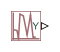# PS Random Number

Generate normally distributed random numbers for physical modeling

•Libraries:
Simscape / Foundation Library / Physical Signals / Sources

## Description

The PS Random Number block generates normally (Gaussian) distributed random numbers. To generate uniformly distributed random numbers, use the PS Uniform Random Number block.

The block behavior is the same as the Simulink® Random Number block (except that it generates a physical signal rather than a Simulink signal) and is based on the polar rejection method (, ).

You have an option to specify an initial time offset as part of the Sample time parameter. In this case, the block outputs 0 until the simulation time reaches the `offset` value, at which point the random sequence starts.

PS Random Number blocks that use the same seed and parameters generate a repeatable sequence. The seed resets to the specified value each time a simulation starts. By default, the block produces a sequence that has a mean of 0 and a variance of 1.

## Ports

### Output

expand all

Output physical signal.

## Parameters

expand all

The mean of the random numbers generated by the block.

The variance of the random numbers generated by the block.

The starting seed for the random number generator. Output is repeatable for a given seed. The seed must be an integer in the range of 0 to (2^32 – 1).

The value of the time `step` interval. The default `step` value is `1` s. To specify an initial time offset, enter the parameter value as ```[step, offset]```, otherwise the `offset` value is assumed to be `0`. The offset must be less than the step size.

 Bell, J. R. “Algorithm 334: Normal random deviates.” Communications of the ACM. Vol. 11, Number 7, 1968, p. 498.

 Knop, R. “Remark on Algorithm 334 [G5]: normal random deviates.” Communications of the ACM. Vol. 12, Number 5, 1969, p. 281.